Welcome To Basics In Maths

# Matrices ( Qns & Solutions) || V.S.A.Q’S||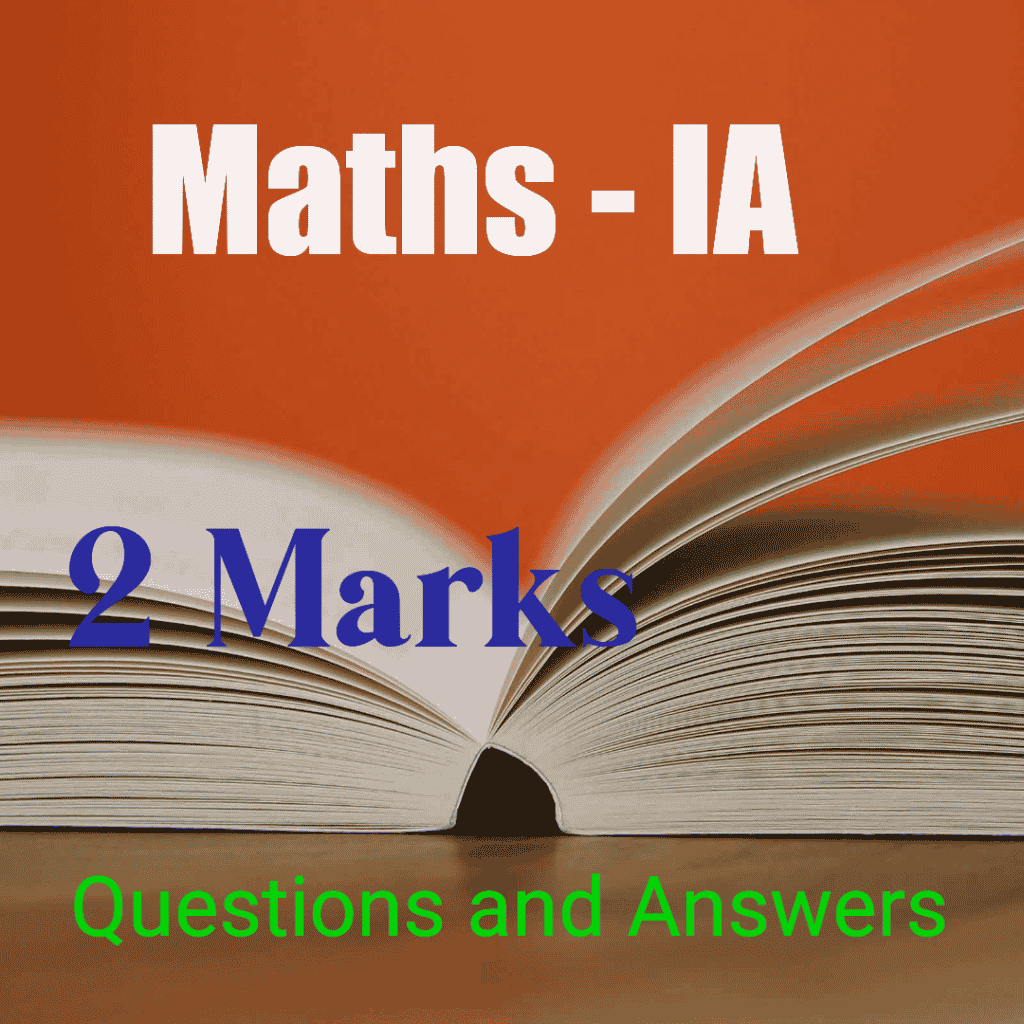# This note is designed by the ‘Basics in Maths’ team. These notes to do help the intermediate First-year Maths students.

### Inter Maths – 1A two marks questions and solutions are very useful in IPE examinations.

Matrices

#### QUESTION 1

If A =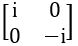, then show that A2 = –I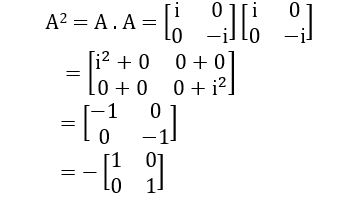∴  A2 = –I

#### QUESTION 2

If A =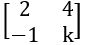, and A2 = 0, then find the value of k.

A2 = 0

8 + 4k = 0, – 2 – k = 0 and –4 + k2 = 0

4k = –8; k = –2; k2 = 4

k = –2; k = –2; k = ± 2

∴ k =– 2

#### QUESTION 3

Find the Trace of A, If A =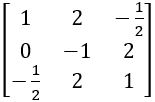Trace of A = 1 – 1 + 1 = 1

##### QUESTION 4

2X = B – A

#### QUESTION 5

Find the additive inverse of A, If A =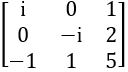Additive inverse of A = – A

##### QUESTION 6

If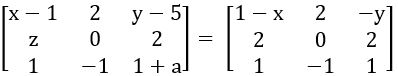, then find the values of x, y, z and a.

⟹ x- 1 = 1 – x ; y – 5 =  – y ; z = 2 ; 1 + a = 1

⟹ x + x = 1 + 1; y + y = 5; z = 2; a =1– 1

⟹ 2x = 1; 2y = 5; z = 2; a = 0

∴ x = ½ ; y = 5/2; z = 2; a = 0

#### QUESTION 7

Construct 3 × 2 matrix whose elements are defined by aij =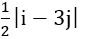Sol:

a11 = 1

a22 = 2

a31 = 0

#### QUESTION 8

If A =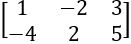and B =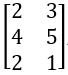, do AB and BA exist? If they exist, find them. BA and AB commutative with respect to multiplication.

Order of A = 2 × 3 and Order of B = 3 × 2

AB and BA exist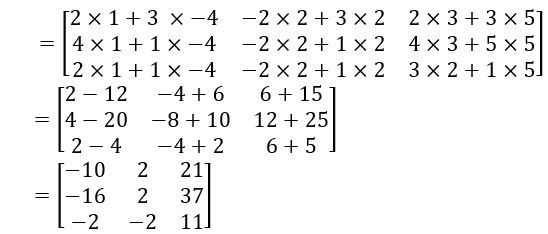AB and  BA are not Commutative under Multiplication

##### QUESTION 9

Define Symmetric and Skew Symmetric Matrices

Sol:

Symmetric Matrix: Let A be any square matrix, if AT = A, then A is called Symmetric Matrix

Skew Symmetric Matrix: Let A be any square matrix if AT = –A, then A is called Skew Symmetric Matrix

###### QUESTION 10

If A =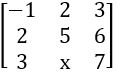is a symmetric matrix, then find x.

Sol: Given, A =is a symmetric matrix

⟹ AT = A

⟹ x = 6

#### QUESTION 11

If A =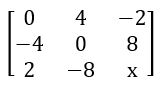is a skew-symmetric matrix, then find x

Sol: Given A =is a skew-symmetric matrix

⟹ AT = – A

⟹ x = –x

x+ x = 0 ⟹ 2x = 0

⟹ x = 0

#### QUESTION 13

##### QUESTION 14

If A =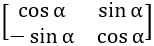, then show that AAT = ATA = I

=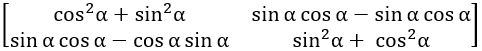∴ AAT = ATA = I

###### QUESTION 15

Find the minor of – 1 and 3 in the matrix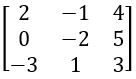Sol: Given Matrix is

minor of – 1 =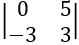= 0 + 15 = 15

minor of 3 =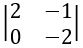= – 4 + 0 = – 4

#### QUESTION 16

Find the cofactors 0f 2, – 5 in the matrix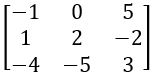Sol: Given matrix is

Cofactor of 2 = (–1)2 + 2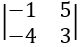= –3 + 20 = 17

Cofactor of – 5 = (–1)3 + 2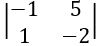= –1(2 – 5) = –1(–3) = 3

#### QUESTION 17

If ω is a complex cube root of unity, then show that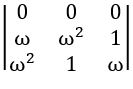= 0(where 1 + ω+ω2 = 0)

R1 → R1 + R2 + R3

##### QUESTION 18

If A =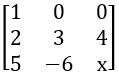and det A = 45, then find x.

Det A = 45

⟹ 1(3x + 24) – 0 (2x – 20) + 0 (– 12 – 15) = 45

⟹ 3x + 24 = 45

3x = 45 – 24

3x = 21

x = 7

###### QUESTION 19

Find the adjoint and inverse of the following matrices

(i)

(ii)

##### QUESTION 20

Find the inverse of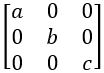(abc ≠ 0)

Det A = a (bc – 0) – 0(0 – 0) + 0(0 – 0)

Det A = abc ≠ 0

Adj A = (Cofactor matrix of A) T

#### QUESTION 20

Find the rank of the following matrices.

Det A = 1 (0 – 2) – 2(1 – 0) + 1(– 1 – 0)

= – 2– 2– 1

= – 5 ≠ 0

∴ Rank of A = 3

Det A = – 1 (24 – 25) + 2(18 – 20) + – 3(15 – 16)

= – 1– 4 + 3

= – 0

Det B = – 4 + 6 = 2 ≠ 0

∴ Rank of A = 2

Det of Sub matrix of A = – 1 – 0 = – 1 ≠ 0

∴ Rank of A = 2

Det of Sub matrix of A =1 (1 – 0) – 0(0 – 0) + 0(0 – 0)

= 1≠ 0

∴ Rank of A = 3